# SQL DISTINCT

## Select with distinct

Redundancy is the repetition of certain data in a table. With the use of DISTINCT clause data redundancy may be avoided. This clause will eliminate the repetitive appearance of same data. DISTINCT can come only once in a given select statement.

Syntax:

```SELECT DISTINCT <column_name>
FROM <table_name>
WHERE <conditions>;
```

Parameters:

Name Description
column_name Name of the column.
table_name Name of the table.
conditions It may be a condition, a select query or an expression.

Pictorial presentation :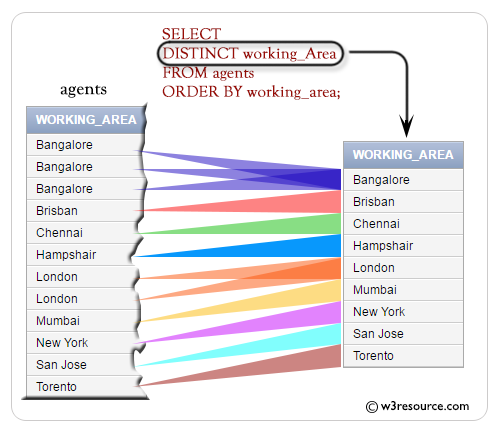Example-1: SQL DISTINCT

Sample table: orders

To get all 'agent_code' from the 'orders' table, the following SQL statement can be used:

SQL Code:

``````SELECT agent_code FROM orders;
``````

Relational Algebra Expression: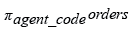Relational Algebra Tree: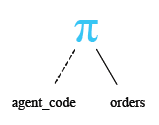Output:

```AGENT_CODE
----------
A008
A004
A006
A010
A004
A011
A005
A013
A004
A005
A011

...
...
```

The above picture shows the same 'agent_code' appears more than once.

Example-2: SQL DISTINCT

To get each 'agent_code' once from the 'orders' table, the following SQL statement can be used :

SQL Code:

``````SELECT DISTINCT agent_code
FROM orders;
``````

Relational Algebra Expression: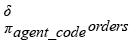Relational Algebra Tree: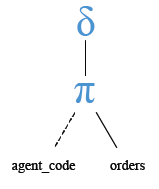Output:

```AGENT_CODE
----------
A004
A002
A007
A009
A011
A012
A010
A013
A001
A008
A006
A005
A003
```

Practice SQL Exercises

﻿

## SQL: Tips of the Day

What is the simplest SQL Query to find the second largest value?

```SELECT MAX(col)
FROM table
WHERE col NOT IN ( SELECT MAX(col)
FROM table
);
```

Ref: https://bit.ly/3jPa662